EMI Suppression Filters (EMC and Noise Suppression)Noise Suppression Products/EMI Suppression Filters

Noise Suppression Basic Course Section 1
Chapter 1
Chapter 2
Chapter 3
Chapter 4
Chapter 5
Chapter 6
Chapter 3

Factors of making noise problems complex

3-3. Noise conduction and reflection

Noise conduction occurs through conductor conduction and spatial conduction. When explaining the nature of conductor conduction, the concept of transmission theory may be used. In order to facilitate your understanding of the following topics, I would like to explain the concept of transmission theory used in EMC in a simplified manner. For detailed and precise explanations, please refer to technical books [Reference 2,3,4] .
The transmission theory considers a conductor as a transmission line, where the electric energy propagates as a wave and reflects at the end of the line. Whether the propagated energy is a signal or noise, it propagates as a wave in the same manner. Therefore, this section first explains the concept of transmission theory with reference to signal instead of noise, and thereby describes the conduction of noise.

3-3-1. Influence of digital signal on pulse waveform

(1) Resonance occurs due to reflection

When digital signal is connected to a relatively long wire of 10cm or more, it may cause a ringing just like the one shown in Fig. 3-3-1. As described in the previous section, this can be explained as resonance due to the inductance and electrostatic capacity inherent in the wiring. However, based on the transmission theory, it can also be considered that the wire itself works as a kind of resonator due to the reflection of signal wave at both ends of the wire as shown in Fig. 3-3-2, making a specific frequency component look prominent. In this way, the transmission theory explains the phenomenon from the viewpoint of conduction and reflection of electric waves.
When the transmission theory is used, the phenomenon of increase in the spectrum (460MHz and 860MHz in the figure) can be predicted not only at the oscillating frequency of the ringing but also in the higher frequency range as shown in Fig. 3-3-1(c).

(2) Reflection interferes with the transmission of signal waveform

If reflection or resonance occurs, the pulse waveform cannot be properly transmitted. In order to correctly transmit the signal form, you need to suppress the reflections at both ends of the wire. If you use the transmission theory, you can provide a design to suppress reflections and predict the change in waveform caused by reflection.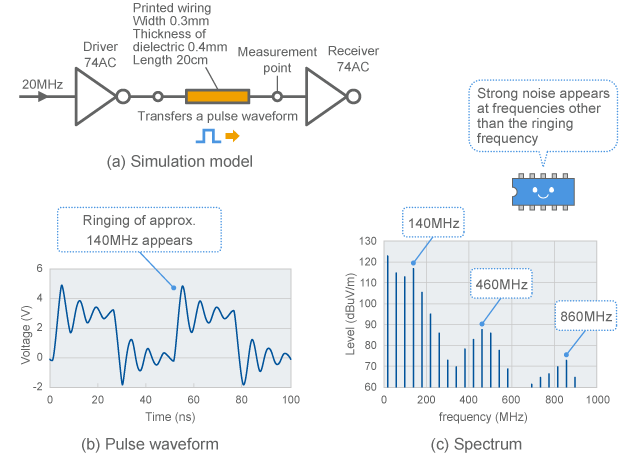Fig. 3-3-1 Example of ringing in digital signal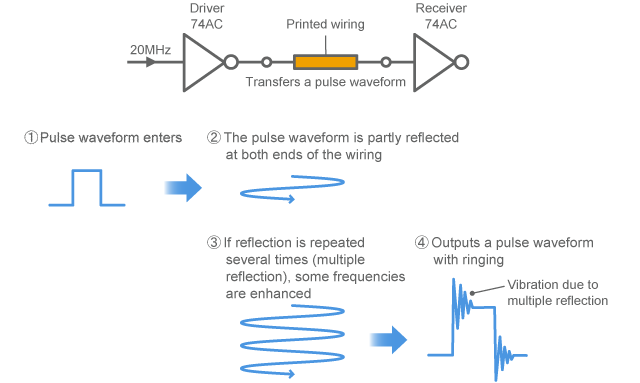Fig. 3-3-2 Mechanism of causing a ringing in digital signal

3-3-2. Characteristic impedance and reflection

(1) Impedance matching

In order to suppress reflections at the ends of wire, "impedance matching" is carried out. The term "matching" refers to matching the "characteristic impedance" of the wire with the "impedance" of the circuit that is connected to the end of the wire.

(2) Characteristic impedance

The conductor that carries the waves of electricity as shown in the signal wiring in Fig. 3-3-3 is called transmission line. When electricity is transmitted through a transmission line, the ratio between electricity and current becomes constant. This ratio is called characteristic impedance. The characteristic impedance is a value determined by the inductance and electrostatic capacity per unit length of wire as shown in Fig. 3-3-3, and it is a pure resistance for a lossless transmission line. When people say 50 ohms or 75 ohms for a coaxial cable, they mean this characteristic impedance. Unless otherwise specified in this text, we consider that transmission lines are ideal and do not have any resistance loss so that the theory and expression can be simplified. This is also applied to the following chapters. (If there is a loss, the characteristic impedance will not be a pure resistance, making the concept complex)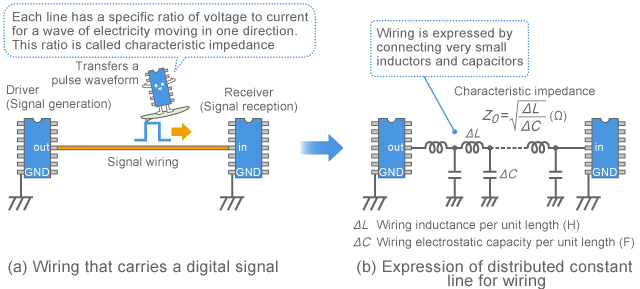Fig. 3-3-3 Distributed constant line model of signal line

As shown in Fig. 3-3-4(b), when the impedance of the circuit (hereinafter, load) connected to the end of wire (hereinafter, terminal) is equal to the characteristic impedance, 100% of the electric energy can be transmitted to the load without any reflection. The signal waveform is also transmitted correctly. In this case, you can say that the terminal of this wire is impedance-matched.

(4) Matching allows transmission of 100% energy

If the end of the wire is attached to another circuit instead of a load, the input impedance of this circuit is regarded as the load impedance to consider the impedance matching. When the input impedance of this circuit is equal to the characteristic impedance of the transmission line, 100% of the energy is transmitted. In this situation, you can say that these two circuits are matched with each other.
In noise suppression, energy transmission is not always a good thing. At the point where the noise transmission path and the noise source or antenna are connected with each other, it is rather advantageous to make a bad impedance matching so as not to transmit the noise energy.

(5) Reflected wave

If the load impedance differs from the characteristic impedance, the signal energy is partly reflected and flows backward through the transmission line as shown in Fig. 3-3-4(c). This wave is called "reflected wave", wherein the magnitude of the reflection is represented by "reflection coefficient." If reflection occurs, a waveform in which the input wave and reflected wave are added is observed at the terminal.

(6) Reflected wave contained in digital signal

Fig. 3-3-5 shows an example of waveform in case that the digital signal is connected with a transmission line and load. As shown in Fig. 3-3-5(a), a 33MHz clock generator signal is transmitted by a 28cm long wire with 50 ohms characteristic impedance.
Fig. 3-3-5(b) shows the case that a load of the same resistance as the characteristic impedance of the wire is connected. The pulse waveform is correctly transmitted. (Since the output resistance of the clock generator is large, there is a rise time of about 2ns)

(7) Digital signal is formed by adding the travelling wave and reflected wave

Fig. 3-3-5(c) shows the case that a digital IC has been connected. The signal amplitude increases while some overshoot and undershoot are observed. This observed waveform is caused by overlaying the reflected wave generated at the terminal over the original signal travelling rightwards. That means the reflected wave with the same sign as the original signal has been generated at the terminal just like Fig. 3-3-4(c), and thus the signal amplitude looks greater than the original signal (Fig. 3-3-5(b)).
As opposed to this case, there is a case that the sing of the reflected wave become opposite to the original signal, making the signal amplitude smaller than the original signal.
The coefficient that represents the sign (more accurately, phase) and magnitude of the reflected wave is the reflection coefficient.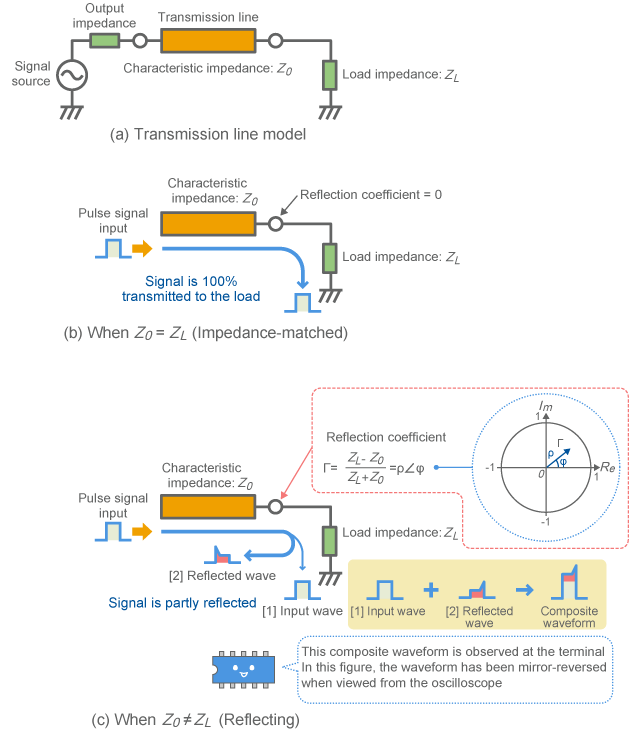Fig. 3-3-4 Signal reflection and matching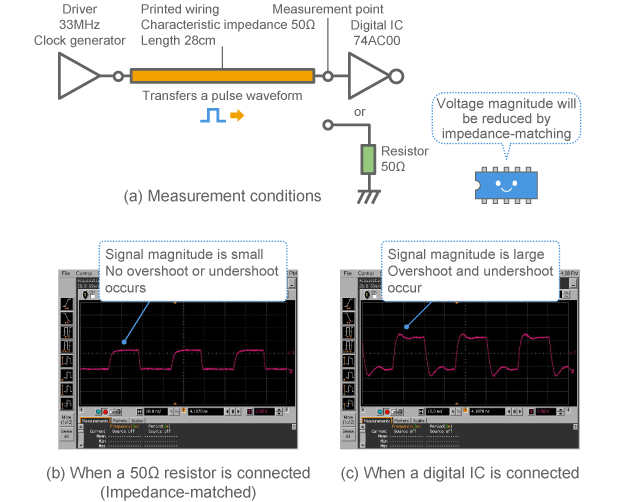Fig. 3-3-5 Example of digital signal waveform when reflection occurs

(8) Reflection coefficient is vector quantity

The reflection coefficient Γ is a vector quantity that has a magnitude ρ and phase angle Φ and can be plotted inside a circle of radius 1 on a complex plane as shown in Fig. 3-3-4(c). Therefore, ρ will have a value between 0 and 1.
ρ =1 means total reflection, while ρ =0 means zero reflection. Usually the value varies depending on the frequency.
As the difference between the characteristic impedance and load impedance becomes greater, the reflection becomes stronger and thus ρ becomes higher (closer to the edge of the circle). In the case of total reflection, ρ is equal to 1, which is plotted on the circumference.

(9) Reflection coefficient at the center of the circle means "matched"

When no reflection occurs (matched), it is plotted at the center of the circle. Representing a reflection coefficient by a position inside the circle as above is useful for intuitively understanding how the status of reflection is. This method is used by the Smith chart.
Inversely, you can calculate the load impedance based on the characteristic impedance and reflection coefficient.
This concept of reflection coefficient is used for S-parameters described later. S-parameters are important concept as those are widely used for electrical measurements of high frequency waves (not limited to noise).

3-3-3. Impedance matching for digital circuit

(1) Characteristic impedance of digital signal

How much is the characteristic impedance of signal lines used for digital circuits? For example, as shown in Fig. 3-3-6, if there is a signal line at the surface of a 4-layered multilayer board that contains a power plane and ground plane as an inner layer, the signal line can be handled as a microstrip line (hereinafter MSL), wherein the characteristic impedance of the signal line is about 50 to 150 ohms. (It can be smaller if there are wires for power supply)

(2) Many digital circuits are not impedance-matched

As opposed to this, the input impedance of digital IC often has a capacitance of several pF, which becomes high impedance of several 100 ohms or more for frequencies of 100MHz and less. Therefore, as shown in Fig. 3-3-7, digital circuits are basically designed to cause extremely high reflection resulting in reflecting most of the signal energy at the receiver.
In addition, the output impedance on the driver side of digital IC also varies. Therefore, matching has not always been achieved on the driver side and is possibly causing reflection. As a result, a digital signal generally causes reflection at both ends of the signal line as shown in Fig. 3-3-2 and is transmitted while causing multiple reflection to some extent.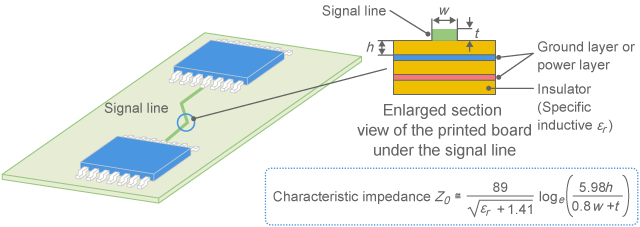Fig. 3-3-6 Characteristic impedance of signal line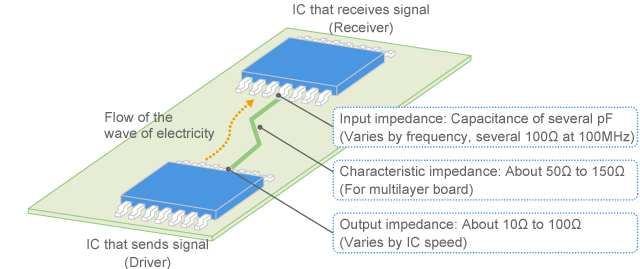Fig. 3-3-7 Impedance matching status of digital signal line

(3) Standing wave indicates the status of matching

Although the input wave and reflected wave were separately described in Fig. 3-3-4 for the sake of explanation, it is difficult to observe those two waves separately in a normal measurement (as an oscilloscope only shows the composite waveform). Therefore the observation of the status of reflection can be carried out by observing the standing wave as described below.
If multiple reflection occurs due to the reflection on both driver and receiver sides, the transmission line forms a kind of resonator making a specific frequency prominent. The resonance by transmission line is not desirable since it causes a ringing from a viewpoint of correctly transmitting a digital signal waveform (called "signal integrity"). In addition, from a viewpoint of EMC, it is also not desirable as it increases noise at the resonance frequency. In order to suppress the resonance by transmission line, both sides or one side of the wire should be brought close to the matched status to absorb the reflection.

3-3-4. Standing wave

(1) Voltage and current appear to be different depending on the measuring point

When measuring noise on a signal line at a certain frequency, if reflection occurs at the terminal, a standing wave is observed as shown in Fig. 3-3-8. This is a phenomenon that you can see a different signal strength depending on the position on the line due to the interference between the "incident wave" (original signal) and reflected wave. This standing wave is the root cause of the complex disposition of transmission line, which will be described hereinafter.
As shown in Fig. 3-3-9, the point showing the maximum amplitude is called "antinode", whereas the minimum point is called "node." The positions of antinode and node vary depending on the frequency. By nature, the position of antinode for voltage becomes a node for current while the position of node for voltage becomes an antinode for current.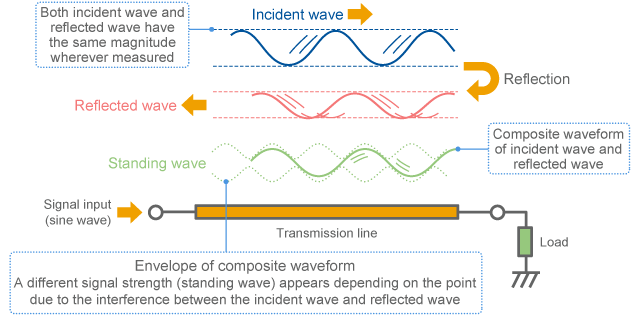Fig. 3-3-8 Standing wave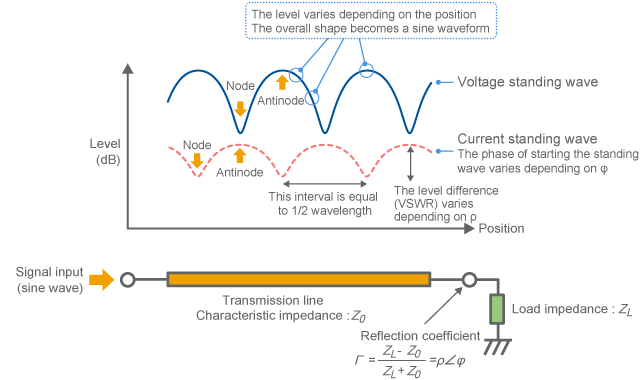Fig. 3-3-9 Voltage standing wave and current standing wave

(2) Observe the standing wave contained in digital signal

Figs. 3-3-10 to 12 show examples of observing the standing wave of the digital signal wave shown in Fig. 3-3-5. Here, a 28cm long signal line is connected to the 33MHz clock signal to observe the magnetic field and electric field around the signal line. The magnetic field and electric field correspond to the current and voltage respectively. The observed frequency is 490MHz (the 15th harmonic of 33MHz clock frequency) and the measurement interval is 5mm.
In each figure, (a) is provided with a 50 ohms resistor at the right end of the signal line to approximately gain impedance matching, while (b) is provided with the input terminal of digital IC.

(3) Current standing wave

Fig. 3-3-11 shows the measurement result of magnetic field. Although (a) with the impedance-matched terminal shows a constant magnetic field over the line, (b) indicates a strong magnetic field (red) and a week magnetic field (blue) depending on the position. That means that the red part has a large electric current. This is called standing wave, wherein a higher reflection coefficient ρ Observe resonance through a standing wave causes a greater difference between maximum and minimum.

(4) Voltage standing wave

Fig. 3-3-12 shows the measurement result of electric field. Just the same as the case of current, (b) with a digital IC as a load indicates variation depending on the position. Comparing Fig. 3-3-11 and Fig. 3-3-12 with each other, you can see that the positions where strong noise is seen are opposite between voltage and current as shown in Fig. 3-3-9.
If a standing wave occurs, the noise level appears to vary depending on the position. Therefore the noise strength cannot be determined just by a single result measured at one position.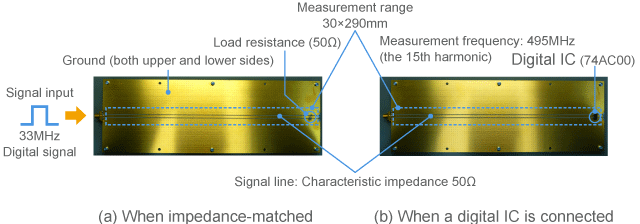Fig. 3-3-10 Measurement range of standing wave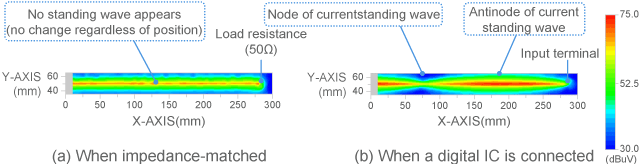Fig. 3-3-11 Measurement result of magnetic field (current)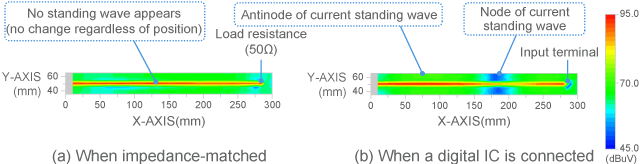Fig. 3-3-12 Measurement result of electric field (voltage)

(5) VSWR

The ratio between the antinode (maximum point) and the node (minimum point) of the voltage standing wave shown in Fig. 3-3-12 is called VSWR (Voltage Standing Wave Ratio), which is an index that represents the extent of reflection. VSWR is disposed to be the same for voltage and current. If there is no standing wave, VSWR is 1. Greater reflection makes VSWR larger. According to the measurement result of the figure, the case (b) in which a standing wave has been observed indicates that the VSWR is about 4.

(6) The cycle of the standing wave is 1/2 wavelength

The length of one cycle (node to node) of this standing wave is indicated to be 1/2 wavelength of the frequency. Since the impedance change and transmission line resonance that will be described later are based on this standing wave, those can repetitively occur at every frequency that makes the length of the transmission line equal to an integral multiple of 1/2 wavelength.
The examples of Fig. 3-3-11 and Fig. 3-3-12 (b) show that one cycle of the standing wave is approx. 200mm, which indicates that one wavelength is 400mm on this line. The wavelength for 490MHz measured in a vacuum condition was approx. 600mm, which indicates that the wavelength has shrunk to 2/3 on this line. This shortening rate is known to vary depending on the specific inductive of the substrate, wherein a greater specific inductive makes the wavelength shorter (that means the wave of electricity slows down on the substrate).

3-3-5. Impedance appears to be different due to transmission line

(1) What makes impedance appear to be different?

Another important nature of signal line from the viewpoint of transmission line is that the load impedance via the line appears to be completely different from what it is.
For example, how does the impedance appear to be at the output terminal of digital IC that is connected to the 20cm long signal line shown in Fig. 3-3-11 In order to find out this, a resistor (10 ohms: Purple, 1000 ohms: Blue), capacitor (5pF: Green) and inductor (50nH: Red) were connected as a load as shown in Fig. 3-3-13 and the impedance were measured. If the digital IC is connected to the terminal port as shown in Fig. 3-3-1, it is considered to be close to the impedance of capacitor (5pF) among those.
Fig 3-3-14 shows the calculation model. (a) shows the case of not considering the signal line, while (b) shows the case of measuring via the transmission line. In addition, just for your reference, (c) shows the case of simulating the wire as a single stage LC circuit as shown in Section 3-2 of Chapter 3.
The calculation results are shown in Fig. 3-3-15. The case (a) of not considering the signal line indicates a constant value without regard to the resistor. Inductor and capacitor respectively show impedances proportional to / inversely proportional to the frequency.

(2) Impedance appears to vibrate due to transmission line

In contrast, the case (b) of considering the transmission line increases the difference from (a) above the frequency of 10MHz and then shows a complex fluctuation above the frequency of 100MHz. If you take a closer look, the impedance seems like vibrating around the characteristic impedance (in this case, 123 ohms) of the signal line as the center.
As above, when you look though the transmission line, the impedance appears to be significantly different in the high frequency range. Although Fig. 3-3-15 only shows the amplitude of the impedance, its phase is also changing. Therefore, depending on the frequency, a inductor can look like a capacitor, and a capacitor can look like a inductor. (In some cases, a transmission line can be used as an impedance converter or used for impedance matching by taking advantage of such characteristics.)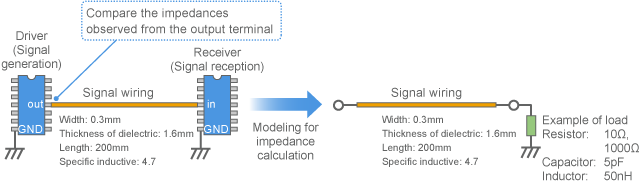Fig. 3-3-13 Impedance observed from the output terminal of digital circuit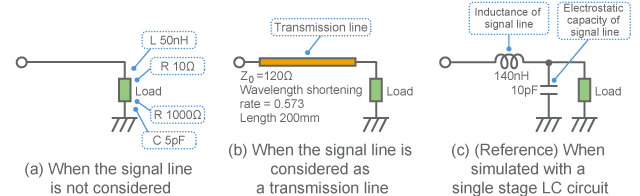Fig. 3-3-14 Calculation model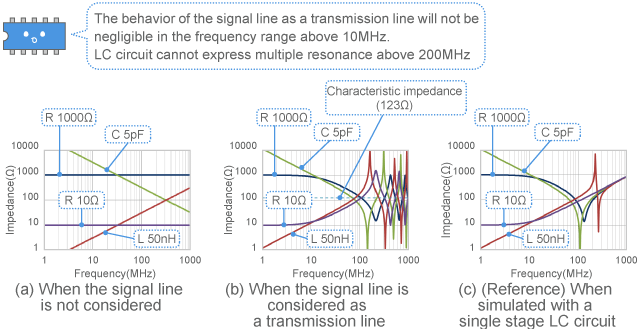Fig. 3-3-15 Impedance comparison

(3) Phase difference between incident wave and reflected wave causes changes in impedance

In the calculation results in Fig. 3-3-15(b), the case of attaching a 5pF capacitor (green line) indicates characteristics that are relatively close to the case of using a digital circuit as a load. This calculation result shows a local minimum impedance point between 100MHz and 200MHz. In the frequency range above 200MHz, a local maximum point and a local minimum point alternatively appear in the impedance showing a cyclic behavior. The frequency interval between a local minimum point and the next local minimum point in impedance will be equal to the frequency that makes the wire length form 1/2 wavelength. As above, the behavior of transmission line has a strong connection with the relationship between the wire length and wavelength.

(4) Be aware of noise at frequencies that cause a local minimum impedance

Since frequencies that cause local minimum impedance allow a large flow of electric current, you need to pay extra attention for the EMC measures. The pulse waveform may cause a ringing, or strong noise may be emitted.

3-3-6. Resonance due to multiple reflection

(1) Transmission line becomes a resonator

If reflection occurs at both ends of the wire as described in digital signal, here appears a frequency at which the wave perfectly fits over the signal of the following cycle as shown in Fig. 3-3-16 while the reflected wave makes one round-trip in the wire. At such a frequency the transmission line may work as a kind of resonator and cause an extremely large voltage or current. Care needs to be taken as it may generate a ringing to the digital signal or cause strong noise at a certain frequency.

(2) Observe resonance through a standing wave

Fig. 3-3-17 adopts the conditions of the 20cm long signal line assumed in Fig. 3-3-14(b) and overlays the calculation results of standing wave at each frequency under the cases that (a) both ends are impedance-matched (no reflected wave), (b) reflection occurs only at the end terminal, and (c) reflection occurs at both ends (multiple reflection). The signal output has been adjusted to be 1V (120dB µ V) under the case of (a).
When no reflection (a) occurs, the voltage is constant (120dB µ V) at the entire frequency range and position. The signal is correctly transmitted under the impedance-matched condition like this.

(3) Standing wave appears when reflection occurs only at one end

The case (b) sets the load impedance to 1Mohm (almost open and total reflection). In this case, a standing wave is observed and the voltage varies depending on the frequency and position. This status is considered to be close to the status measured in Fig. 3-3-11 and Fig. 3-3-12. If reflection occurs only at one end, the maximum value will not exceed two times (increase by 6dB) the original signal regardless of how hard the reflection may be.

(4) Large vibration appears at the resonance frequency when reflection occurs at both ends

The case (c) causes reflection by reducing the output impedance of the signal source to 10 ohms in addition to the terminal conditions of (b). In this case, an extremely strong standing wave has been observed at some frequencies (approx. 200MHz and 650MHz). These frequencies are causing multiple reflection and in some cases the voltage or current may reach several times the original signal and thus can be a problem in terms of EMC measures.

(5) Resonating transmission line also works as an antenna

When the signal line works as a resonator as described above, you need to be cautious since the signal line itself may become a kind of patch antenna and emit strong noise. The resonance frequencies are disposed to repetitively appear at the frequency interval that makes the wire length form 1/2 wavelength (approx. 400MHz in the example of Fig. 3-3-17). Care needs to be taken not to cause the harmonics of the digital signal to come to these frequencies.
In order to avoid resonance due to multiple reflection, the impedance matching shall be carried out at both ends or at one end as shown in Fig. 3-3-17(a) or (b) so as to absorb the reflection. How to terminate the line for digital signal will be described in the next section.
Apart from such a signal circuit, if dealing a conduction path for noise (for example, power line), it is often OK to attenuate the signal. In this case, the resonance can be avoided by increasing the attenuation of the transmission line in addition to the termination. To increase the attenuation, a resistance component is generally added.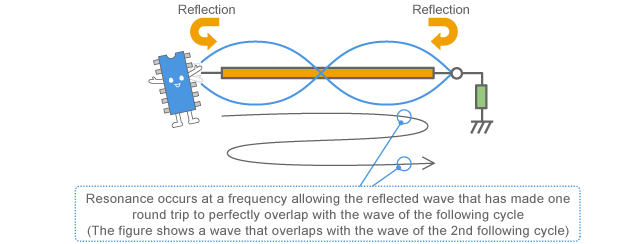Fig. 3-3-16 Resonance by multiple reflection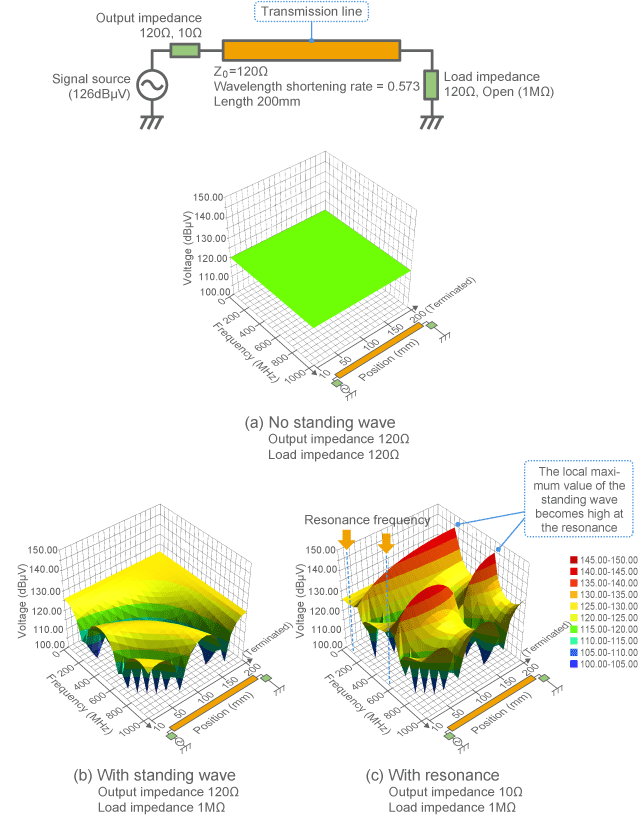Fig. 3-3-17 Changes in standing wave due to resonance

3-3-7. Termination of digital signal

(1) Longer wire also requires impedance matching for digital signal

As described above, when the characteristic impedance of the transmission line is equal to the load impedance, all energy is transferred to the load without reflection. This status is called "matching." For example, Fig 3-3-11(a) and Fig. 3-3-12(a) have a 50 ohms resistor at the end of the signal line so that the characteristic impedance (50 ohms) can be matched. In this case, the electric field (voltage) and magnetic field (current) are uniform and no standing wave is observed.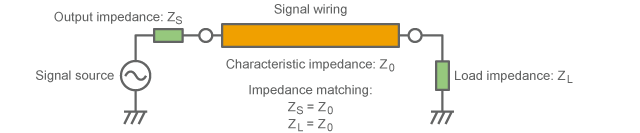Fig. 3-3-18 Impedance matching

In case of digital signal, when C-MOS ICs are connected with each other, both ends of the signal line usually cause reflection. However, if the wiring is short, the resonance frequency will be extremely high and will not cause any practical problem. However, if the wiring is getting longer, the resonance frequency will be lowered and influential and thus may require matching. Impedance matching may be performed on the driver side or on the receiver side as shown in Fig. 3-3-19.

(2) When impedance matching on the driver side

When matching on the driver side Fig. 3-3-19(a), a resistor or ferrite bead is attached in series with the signal line. This is the same as the damping resistor for circuit. The only difference is how to choose the value of resistance. The value of resistance is chosen to the fill the difference between the driver's output resistance and the characteristic impedance. In this case, the receiver side remains to cause reflection and thus a standing wave stays on the signal line deforming the waveform in the middle section of the wiring. Therefore, this is suitable for one-to-one signal transmission that does not have any circuit connected in the middle section of the wiring.

(3) When impedance matching on the receiver side

When matching on the receiver side Fig. 3-3-19(b), a resistor that is equal to the characteristic impedance is connected to the ground or power supply as shown in the figure. In this case, no standing wave is caused and thus a clean pulse waveform can be obtained even if the signal is picked up from the middle section of the wiring. However, this matching has disadvantages of e.g. reducing the signal amplitude and causing power loss due to the current flowing into the load resistor. In order to reduce the power loss in a stationary state, a capacitor may be inserted in series with the resistor.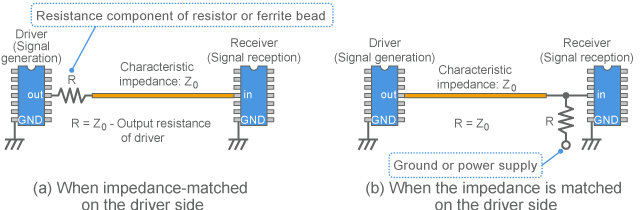Fig. 3-3-19 Impedance matching used for digital circuit

3-3-8. Influence on EMC measures

Although the occurrence of standing wave and resonance are unwanted phenomena in terms of transmitting a digital signal, these are important characteristics that should be considered when investigating noise conduction and considering countermeasures. When the target noise frequency increases, you need to consider measurements and EMC measures based on the assumption that the noise conduction path behaves like a transmission line (causing a standing wave). Examples of the major influences are described below.

(1) Voltage and current appear to be different depending on the measurement point

When looking for a noise source with a probe for EMC measures, one section has stronger noise while other sections have weaker noise even on the same wiring. In addition, the position where strong noise appears is different between voltage and current (magnetic field). Therefore, if the measurement point is different before and after the noise suppression, you cannot correctly evaluate the effect.
Fig. 3-3-20 shows the change in spectrum as an example of this change when moving the measurement point with use of the system of measurement shown in Fig. 3-3-10. When moving the probe by several centimeters, you can see that the shape and level of the spectrum appear to change. If you look for a section with strong noise, you need to keep this change in mind and take measurements at plurality of points to determine the strength.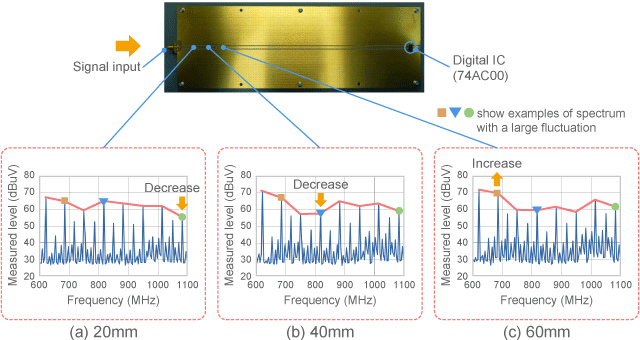Fig. 3-3-20 Example of change in spectrum from point to point

(2) Impedance and the effect of EMC measure component change from point to point

When a standing wave occurs, the impedance is high at the antinode of voltage (node of current) while it is low at the node of voltage (antinode of current). The high or low of this impedance influences the effect of the EMC measure component attached to the position. (However, the shape of the standing wave varies depending on the frequency. Therefore, you cannot generally identify advantageous or disadvantageous positions for all frequencies when attaching an EMC measure component.)
As an example, Fig. 3-3-21 shows the change of the current standing wave shown in Fig. 3-3-11 depending on the frequency. The impedance is smaller where the current is large (relatively red), while the impedance is larger where the current is small (relatively blue). You can see that these positions move in accordance with the frequency.
Generally bypass capacitors tend to have smaller effects at positions where the impedance becomes minimum (current antinode). Fig. 3-3-9 indicates such a position with an arrow. If a component is placed at the position, the effects are reduced for the frequency and thus you need to additionally use a ferrite bead etc. (You may move the position. But you might have a problem at another frequency)
In contrast, ferrite beads may have smaller effects at the local maximum point of the impedance.
An LC filter that combines a capacitor and ferrite bead tends to be relatively less susceptible to the impedance fluctuation in terms of the noise reduction effect.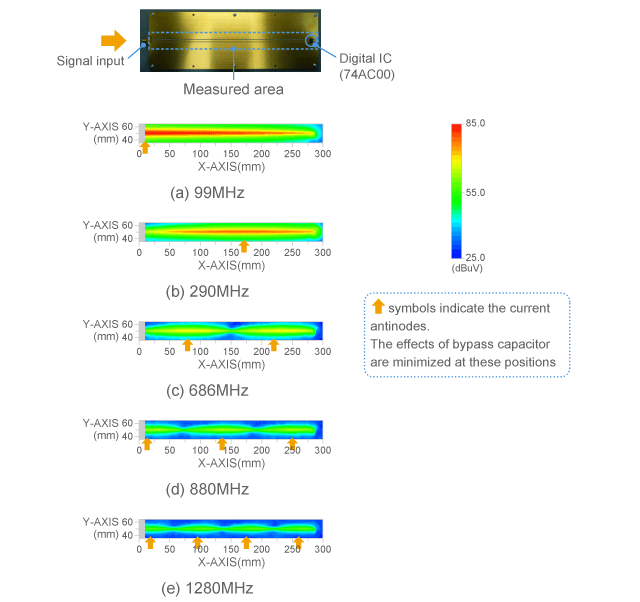Fig. 3-3-21 Example of change in standing wave by frequency

(3) Resonance frequency varies depending on the wiring length

Since a frequency at which the transmission line resonates causes a large voltage and current, it tends to cause a strong noise emission. This frequency varies depending on the length of wiring. Therefore, if you change the wiring length due to relocation of the IC as shown in the figure, it might increase noise at an unexpected frequency. It is hard to predict this type of failure since circuit diagrams do not usually specify the wiring length.
In addition to the signal line, the power supply pattern, cable and shield surface may form a transmission line and cause a resonance. This type of resonator works as a good antenna to emit noise.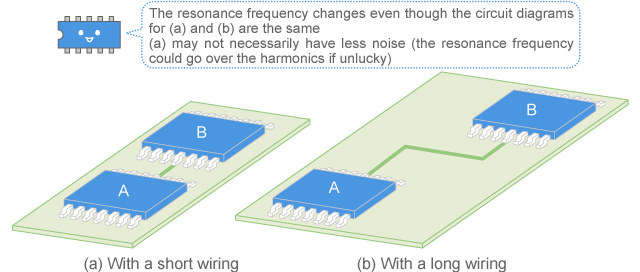Fig. 3-3-22 Resonance changes as the wiring length changes

(4) Standing wave is induced to a cable or shield plate, which in turn works as a good antenna

Regarding the mechanism that a cable connected to an electronic device or a metal plate used in a device works as an antenna, such a conductor can be considered to work as a transmission line causing a resonance. (However, the characteristic impedance of antenna is generally not a constant)
For example, as shown in Fig. 3-3-23, when an electronic device is attached to a cable that has an open end, the cable can be considered as a transmission line that has an open end. In this case, the cable has a standing wave that has zero current at the end part as shown in the figure. Therefore the impedance is reduced at the base part and the current flows without connecting anything at the end part. The resonant condition occurs at frequencies that the cable length is equal to 1/4 wavelength multiplied by an odd number, and thus noise is likely to be emitted.
In this case, the base part has smaller impedance, and thus noise can be controlled by a component that increases impedance such as a ferrite core etc.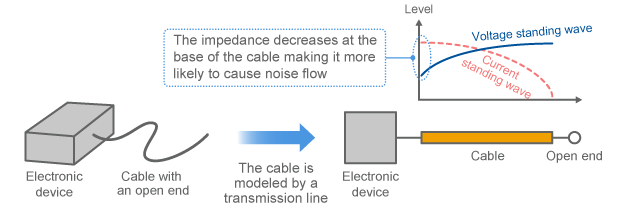Fig. 3-3-23 Electric current occurs on a cable with an open end

As shown in Fig. 3-3-24, if there is a metal plate that is connected to the ground at one end (when a shield plate is connected at one end), standing waves that have zero voltage at the grounded part occur. The frequencies at which the length of the metal plate is equal to 1/4 wavelength multiplied by an odd number cause a resonance and are likely to cause noise emission and induction. If both sides are connected to the ground, standing waves that have zero voltage at both ends occur, and thus the frequencies at which the length of the metal plate is equal to 1/2 wavelength multiplied by an integer cause a resonance. In order to eliminate such a failure, the interval between the points connected to the ground are reduced to about 1/10 or less of the noise wavelength.
As described above, in a (relatively high) frequency range wherein the size of the conductor used for an electronic device exceeds 1/4 wavelength (for example, 750MHz for 10cm), the conductor is likely to work as an antenna. If the frequency of the target noise is high, you need be aware of the relationship between the object size and the wavelength.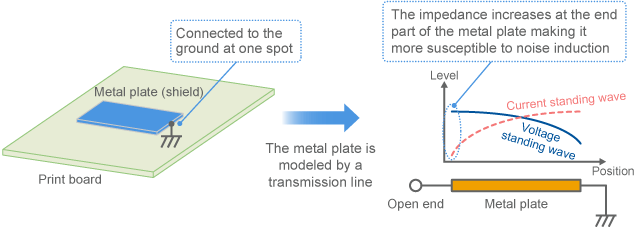Fig. 3-3-24 Metal plate connected to the ground at its end works as an antenna

3-3-9. How to prevent noise conduction

(1) Impedance mismatch can prevent noise conduction

Achieving impedance matching does not necessarily always provide good results. If you prevent noise conduction (instead of signal transmission), you need to avoid matching.
As described in Section 2-1., departing from a broad understanding of the mechanism of noise emission from electronic devices, you can think that a noise transmission path has been created from the noise source origin to the antenna as shown in Fig. 3-3-25. In this situation, if the impedance has been well matched, noise can be conducted to the antenna and cause a strong emission.

(2) Decoupling capacitor causes impedance mismatch

In order to prevent noise conduction, the reflection on both sides of the transmission line should be increased so that noise cannot be conducted. In so doing, a decoupling capacitor or inductor etc. is used to significantly change the impedance to increase the reflection.
It is also effective to increase the attenuation of the transmission path. In order to increase the attenuation, the energy needs to be absorbed. That is why EMC measure components are desirable to exert a noise absorption effect. Ferrite beads that have resistive impedance are effective.
Although Fig. 3-3-25 simply refers to noise transmission path for everything, it is actually a combination of many transmission paths. For example, if power supply noise from a digital IC is emitted from the interface cable, it can be considered as shown in Fig. 3-3-26 (as an example). The reflection and attenuation of noise shown in Fig. 3-3-25 can be applied by disassembling the transmission path into each type.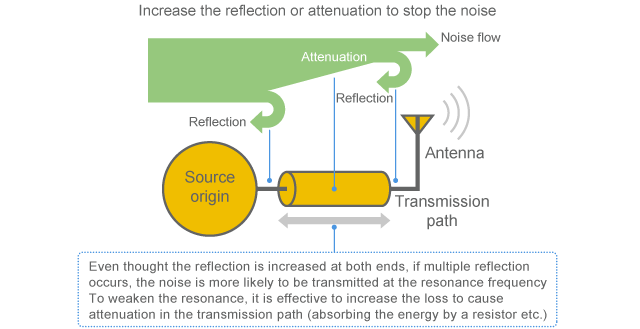Fig. 3-3-25 Noise reflection and attenuation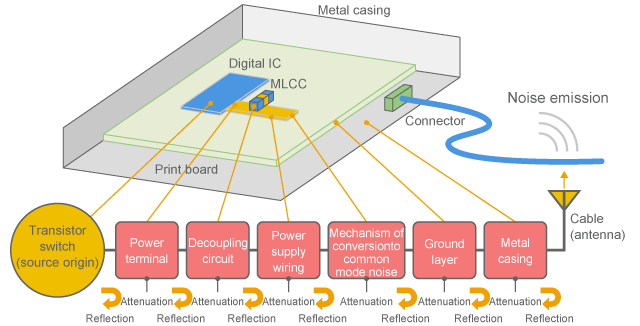Fig. 3-3-26 Example of disassembled noise transmission path

3-3-10. S-parameter

(1) Performance of EMC measure component can be expressed by S-parameters

Although the effects of an EMC measure component used in a noise transmission path are generally expressed by insertion loss, S-parameters are used for more precise expression. The S-parameter method is a way to express the characteristics of a circuit with use of the concept of the electric wave reflection explained above. Since it is able to express the performance of component up to the high frequency, it is often used for high-frequency circuits.

(2) Insertion loss characteristics can be substituted by S-parameters

When expressing an EMC measure component by S-parameters, the insertion loss that represents the noise reduction performance can be substituted by the S-parameter transmission coefficient. This is on the premise that the circuit is linearly operated, and S-parameters that have been measured on 50 ohms system are used.

(3) Transmission coefficient, reflection coefficient

The S parameters of an component that has one input terminal and one output terminal (also called "port") are represented by transmission coefficient and reflection coefficient obtained by inputting an electric wave from the left and right sides as shown in Fig. 3-3-27. The attenuation inside the component explained in Fig. 3-3-25 is the amount of the input energy subtracted by the transmitted portion and reflected portion.

(4) Expression by a table of figures

Since the S-parameters generally change as the frequency changes, those are provided in a table form with values for each frequency. As an example of S-parameters, Fig. 3-3-28 shows the S-parameters for a three-terminal EMC measure component, NFE61PT102. This is an EMC measure component with a relatively large attenuation inside the component.
The figure on the left is a table of S-parameters. As shown in the table, the reflection coefficients and transmission coefficients of each port are indicated by magnitude and phase. (In some cases, those are expressed by real number and imaginary number, or the magnitude may be expressed in dB)

(5) Graph of frequency characteristics

The figure on the right side plots the transmission coefficient S21 and reflection coefficient S11 as frequency characteristics. The transmission coefficient S21 is large in the low frequency range, while it is very low above 10MHz. This characteristic represents the ratio of the noise entered from the left and transmitted to the right, and a smaller value indicates a better noise reduction performance. In order to convert it into insertion loss, the magnitude is converted into dB without the negative sign.
The reflection coefficient S11 is indicated to be around 0.2 to 0.6 in the range from 1MHz to 1GHz. This characteristic indicates the ratio of the reflection going back to the noise source when noise enters from the left. You can see that this component has relatively small reflection and is less likely to cause failure by multiple reflection.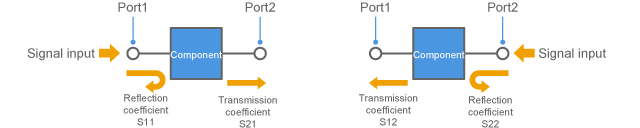Fig. 3-3-27 S-parameters (for 2 port component)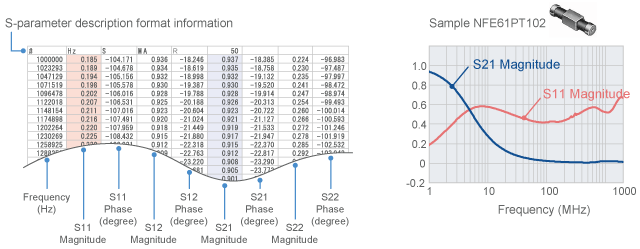Fig. 3-3-28 Example of S-parameters (NFE61PT102)

(6) Advantages of expressing characteristics in S-parameters

When an EMC measure component is expressed with use of S-parameters, you can express not only the primary noise reduction effect (transmission coefficient) but also the effects of the reflection to the noise source side so that you can consider the secondary effects due to multiple reflection. From this viewpoint, S-parameters allow more precise expression than insertion loss does.
S-parameters change when the impedance of the measurement system changes. Usually it is measured in 50 ohms system. In order to accurately estimate the noise reduction effect, you need to interpret it through conversion depending on the impedance of the section where the actual component is attached. Usually circuit simulators are equipped with such a function.
In addition to the graph in Fig. 3-3-28(b), S-parameters can be expressed as plots on the Smith chart as shown in Fig. 3-3-4(c).

“3-3. Noise conduction and reflection” - Key points

• The transmission theory considers that electricity propagates as a wave and reflects
• Any deviation between the characteristic impedance of wiring and the load impedance causes reflection
• Reflection causes a standing wave on the wiring and thus the impedance appears to be different or resonance occurs
• This resonance repetitively occurs at the frequency interval that makes the wire length form 1/2 wavelength
• In order to stop noise conduction, there are two methods: large reflection and internal attenuation
• Component characteristics can be expressed by S-parameters